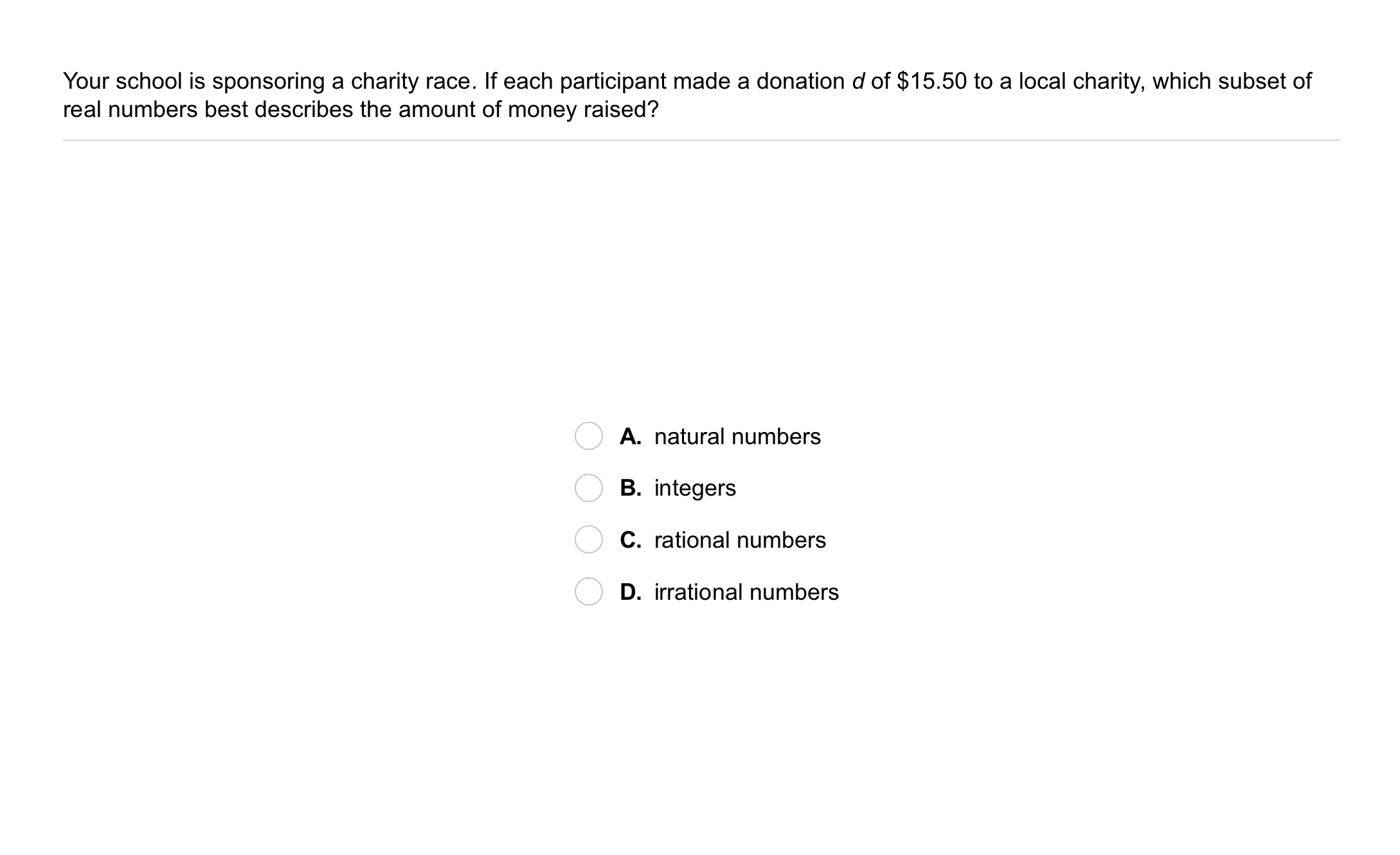Algebra 2 1-2 Guided Practice: Properties of Real Numbers
starstarstarstarstarstarstarstarstarstar
by Matthew Richardson
| 7 Questions1
1
10
Solve It! How can you describe a set that includes five of the emoticons shown, but not the sixth?2
2
10
Problem 1 Got It?
A
B
C
D
3
10
Problem 2 Got It? Graph and label each of the numbers on the number line provided on the canvas. Use a color other than black.

4
10
Problem 3 Got It? Fill in the blank to compare the two numbers below.

>
=
<
5
10
Problem 3 Got It? Reasoning: Let a, b, and c be real numbers such that a < b and b < c. How do a and c compare?
a < c
There is not enough information to compare a and c.
a = c
a > c6
10
Problem 4 Got It? Which property does the equation illustrate?

Associative property
Distributive property
Commutative property
7
10
Problem 4 Got It? Use properties of real numbers to show that the equation is true. Show and justify each step in your process on the canvas provided. Hint: In this item, you will simplify the left side of the equation.
You may use the typing or drawing tools or both as you work.